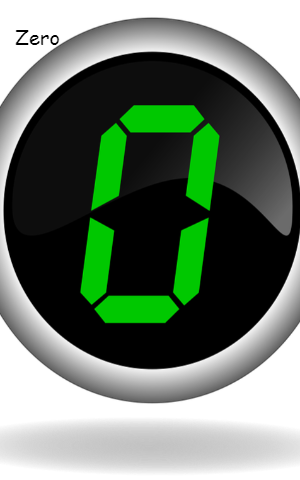#### Zero1 min418

I am a magic number

Value-less when alone

Priceless when the right of any positive number

Right to my positive

Left to me is negative

Neither positive nor negative I am

If you add me or subtract to any number

Number remained unchanged

But if you subtract a positive from me, becomes negative

If you multiply or divide with me any number

The resultant number becomes zero

Neither Prime nor Composite

Alone I am a digit

With any number, I am additive if on the right side

I am ornamental if on the left side

Lonely even not positive

Only natural number but not positive

Smallest integer but not negative

I am not only zero

Science without me is zero

I am a hero

I am zero